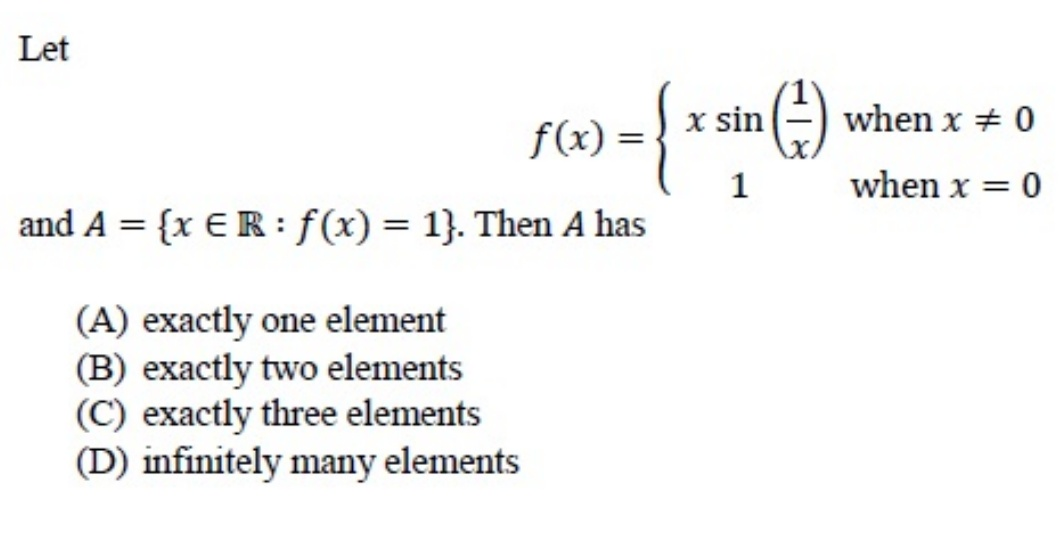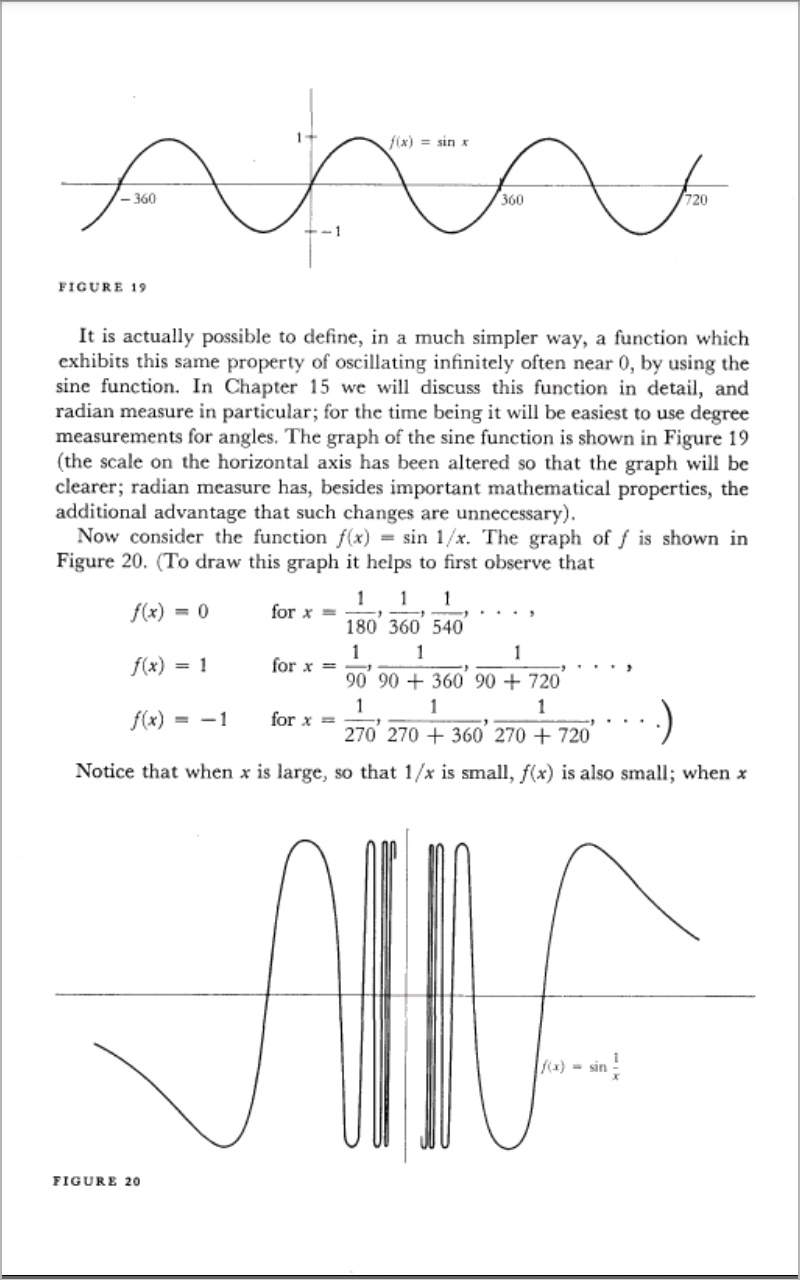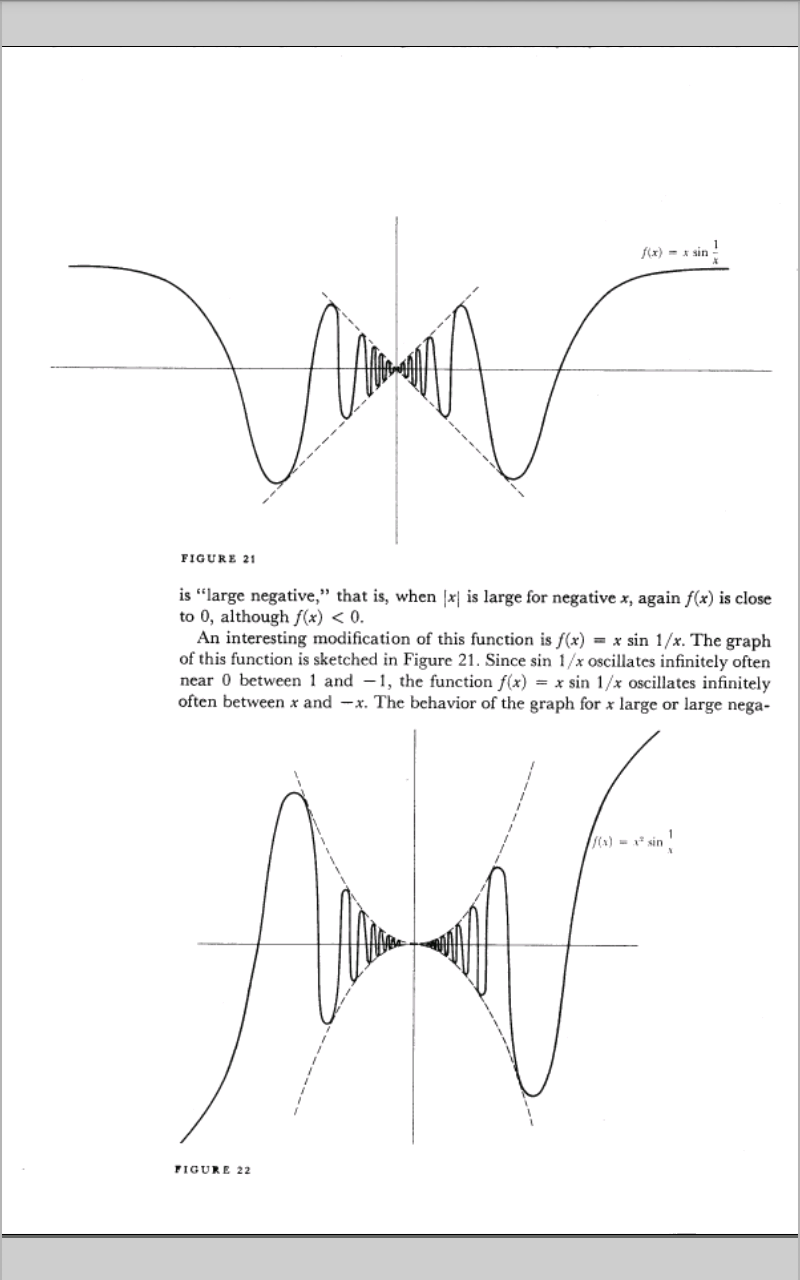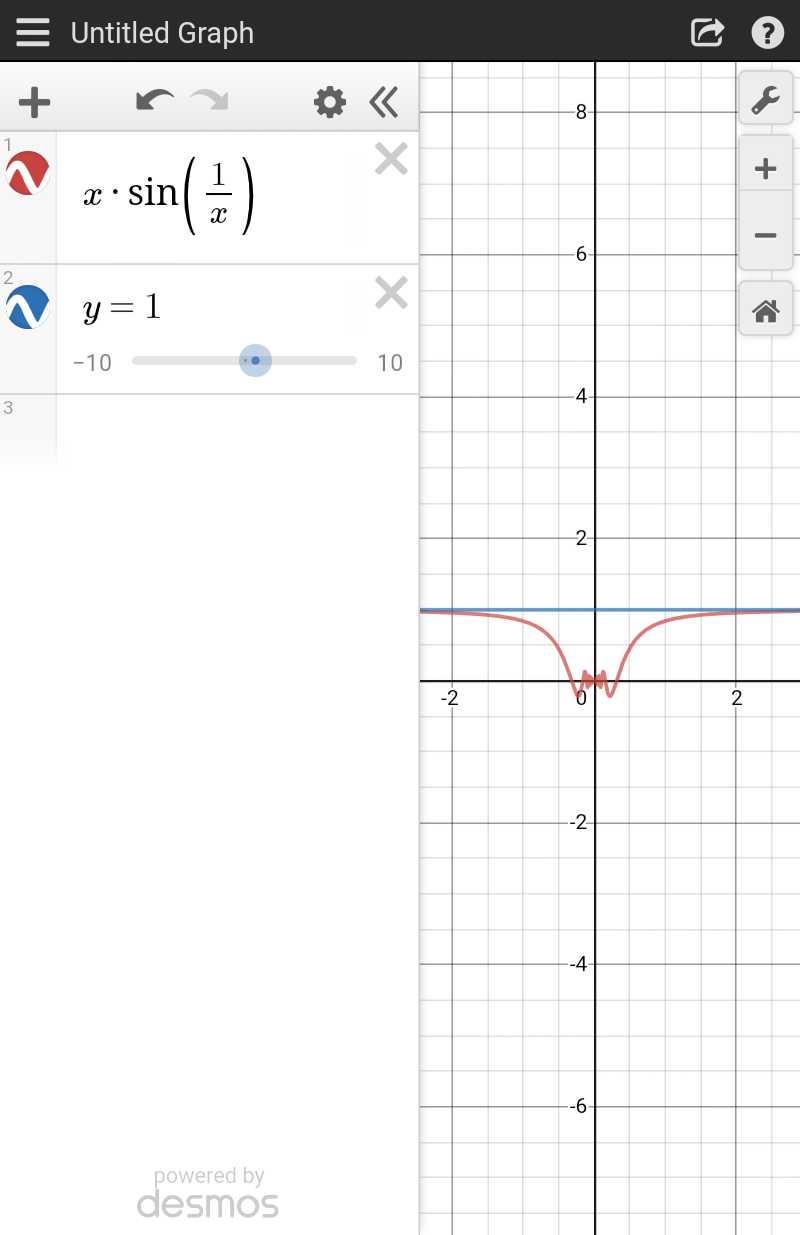# Doubt from Differential calculusIs option A correct ?

0 elements
check
https://www.desmos.com/calculator/t1wrjnemvt

KVPY question right? Wasn't it option B acc to their answer key?

@Rachit_2020 there would be no solution for xsin(1/x) =1
But for x=0 it is given f(0)=1 and hence 1 solution should be the answer

Infact "Exacty one element" seems to be a very straightforward answer.... wondering why it was given option B in the key!

@ananda_2018 are you sure that answer was option B ? Because for xsin(1/x) =1
Sin(1/x) = 1/x, let 1/x = x’ then sinx’= x’
Drawing graph of both of these we get x’ = 0 as the only solution and thus x’ = 0 = 1/x but 1/x can never be zero

It is not solution but graph...@Shwetanshu_2018 ofc yeah it should be "exactly one solution imo as well" but the answer key had a different answer as far as i could remember.

x.sin(1/x)=1 when x= +ve infinity and -ve infinity so two solution...it is my opinion...

From graph i concluded..

But @Tanmay_1 for x=0 ,we already have a solution...this would mean 3 solutions from yur approach! But B is the answer given!

@Tanmay_1 infinity is never calculated in number of solutions , also limiting value at infinity will be 1 so it will never be exactly 1
And at zero it is given that f(x)=1 and hence 0 is for sure an answer

Yes you are correct ...let me explore further...